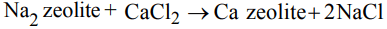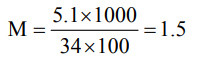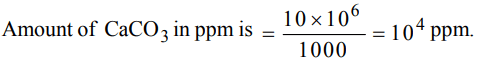## Hydrogen Questions and Answers Part-5

1. When zeolite (hydrated sodium aluminium silicate) is treated with hard water the sodium ions are exchanged with
a) $H^{+}ions$
b) $Ca^{2+}ions$
c) $SO_4^{2-} ions$
d) $OH^{-}ions$

Explanation:2. The species that does not contain peroxide ions
a) $PbO_{2}$
b) $H_{2}O_{2}$
c) $SrO_{2}$
d) $BaO_{2}$

Explanation:3. Hydrogen peroxide does not
a) liberate iodide from KI
b) turn titanium salt yellow
c) gives silver peroxide with moist silver oxide
d) turn mixture of aniline, $KClO_{3}$ and dil. $H_{2}SO_{4}$ violet

Explanation: No such reaction is known (c).

4. 30 volume hydrogen peroxide means
a) 30% of $H_{2}O_{2}$ solution
b) $30cm^{3}$ solution contains 1g of $H_{2}O_{2}$
c) $1cm^{3}$ of solution liberates 30 cm3 of O2 at STP
d) $30cm^{3}$ of solution contains 1 mole of $H_{2}O_{2}$

Explanation: 30 vol of H2O2 means one volume of H2O2 on decomposition will give 30 volume of oxygen

5. The molarity of a 100 ml solution containing 5.1 g of hydrogen peroxide is
a) 0.15 M
b) 1.5 M
c) 3.0 M
d) 50.0 M

Explanation:6. The oxidation states of most electronegative element in the products of reaction $BaO_{2}$ with dil. $H_{2}SO_{4}$  are
a) 0 and – 1
b) –1 and – 2
c) – 2 and 0
d) – 2 and + 1

Explanation:7. Permanent hardness of water can be removed by adding Calgon $\left(NaPO_{3}\right)_{n}$  . This is an example of
b) exchange of ion
c) precipitation
d) none of these

Explanation: There is exchange of ions

8. Water contracts on heating
a) to 100°C
b) from 0°C to 4°C
c) to 273 K
d) from 10°C to 20°C

Explanation:9. 1000g aqueous solution of $CaCO_{3}$ contains 10g of calcium carbonate . Hardness of solution is
a) 10 ppm
b) 100 ppm
c) 1000 ppm
d) 10000 ppm